# Class 12 Maths NCERT Solutions for Chapter 9 Differential Equations Exercise 9.2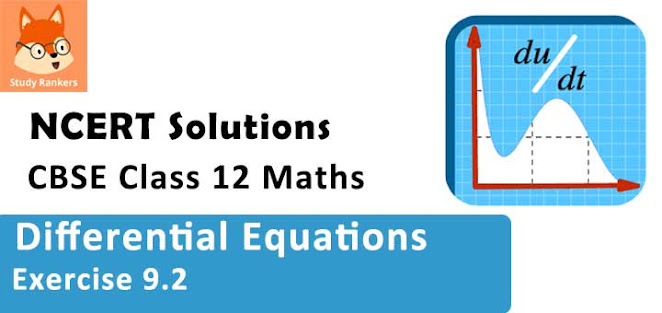### Differential Equations Exercise 9.2 Solutions

1. Verify that the given functions (explicit or implicit) is a solution of the corresponding differential equation
y = ex + 1 : y″ – y′ = 0

Solution

y = ex + 1
Differentiating both sides of this equation with respect to x, we get :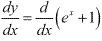⇒ y' = ex ...(1)
Now, differentiating equation (1) with respect to x, we get :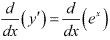⇒ y' = ex
Substituting the values of y' and y'' in the given differential equation, we get the L.H.S. as :
y'' - y' = ex - ex = 0 R.H.S.
Thus, the given function is the solution of the corresponding differential equation.

2. verify that the given functions (explicit or implicit) is a solution of the corresponding differential equation
y = x2 + 2x + C : y′ – 2x – 2 = 0

Solution

y = x2 + 2x + C
Differentiating both sides of this equation with respect to x, we get :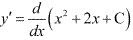⇒ y' = 2x + 2
Substituting the value of y' in the given differential equation, we get :
L.H.S = y' - 2x - 2 = 2x + 2 - 2x - 2 = 0 R.H.S.
Hence, the given function is the solution of the corresponding differential equation.

3. verify that the given functions (explicit or implicit) is a solution of the corresponding differential equation
y = cos x + C : y′ + sin x = 0

Solution

y = cos x + C
Differentiating both sides of this equation with respect to x, we get :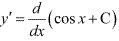⇒ y' = - sin x
Substituting the value of y' in the given differential equation, we get :
L.H.S. = y' + sin x = - sin x + sin x = 0 = R.H.S.
Hence, the given function is the solution of the corresponding differential equation.

4. Verify that the given functions (explicit of implicit) is a solution of the corresponding differential equation y = √(1 + x2) : y' = xy/(1+ x2)

Solution

y = √(1 + x2 )
Differentiating both sides of the equation with respect to x, we get :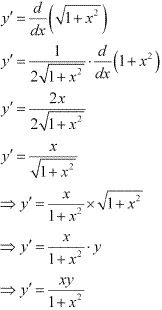∴ L.H.S. = R.H.S.
Hence, the given function is the solution of the corresponding differential equation.

5. verify that the given functions (explicit or implicit) is a solution of the corresponding differential equation
y = Ax : xy′ = y (x ≠ 0)

Solution

y = Ax
Differentiating both sides with respect to x, we get :
y' = d/dx (Ax)
⇒ y' = A
Substituting the value of y' in the given differential equation we get :
L.H.S. = xy' = x.A = Ax = y = R.H.S
Hence, the given function is the solution of the corresponding differential equation.

6. verify that the given functions (explicit or implicit) is a solution of the corresponding differential equation y = x sin x : xy′ = y + x√(x2 - y2 ) (x ≠ 0 and x > y, or x < -y)

Solution

y = x sin x
Differentiating both sides of this equation with respect to x, we get :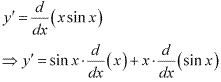⇒ y' = sin x + x cosx
Substituting the value of y' in the given differential equation, we get :
L.H.S = xy' = x(sin x + x cos x)
= x sin x + x2 cos x
= y + x2 .√(1 - sin2 x)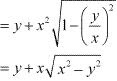= R.H.S
Hence, the given function is the solution of the corresponding differential equation.

7. verify that the given functions (explicit or implicit) is a solution of the corresponding differential equation xy = log y + C : y' = y2/(1 - xy)(xy ≠ 1)

Solution

xy = log y + C
Differentiating both sides of this equation with respect to x, we get :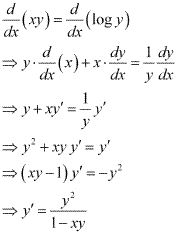∴ L.H.S = R.H.S
Hence, the given function is the solution of the corresponding differential equation.

8. verify that the given functions (explicit or implicit) is a solution of the corresponding differential equation:
y – cos y = x : (y sin y + cos y + x) y′ = y

Solution

y - cos y = x ...(1)
Differentiating both sides of the equation with respect to x, we get :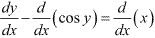⇒ y' + sin y . y' = 1
⇒ y'(1 + sin y( = 1
⇒ y' = 1/(1 + sin y)
Substituting the value of y' in equation (1), we get :
L.H.S = (y sin y + cos y + x)y'
(y sin y + cos y + y - cos y) × ([1/(1 + siny)]
= y(1 + sin y). 1/(1 + sin y)
= y
= R.H.S.
Hence, the given function is the solution of the corresponding differential equation.

9. Verify that the given functions (explicit or implicit) is a solution of the corresponding differential equation
x + y = tan–1y : y2 y′ + y2 + 1 = 0

Solution

x + y = tan-1 y
Differentiating both sides of this equation with respect to x, we get: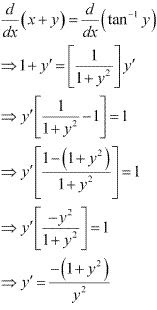Substituting the value of y' in the given differential equation, we get :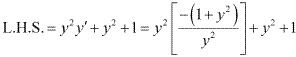= -1 - y2 + y2 + 1
= 0
= R.H.S.
Hence, the given function is the solution of the corresponding differential equation.

10. verify that the given functions (explicit or implicit) is a solution of the corresponding differential equation
y = √(a2 - x2 x) ∈ (-a, a) : x + y (dy/dx) = 0 (y ≠ 0)

Solution

y = √(a2 - x2 )
Differentiating both sides of this equation with respect to x, we get :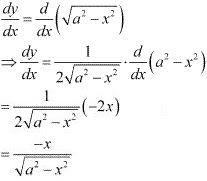Substituting the value of dy/dx in the given differential equation, we get :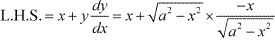= x - x
= 0
= R.H.S
Hence, the given function is the solution of the corresponding differential equation.

11. The numbers of arbitrary constants in the general solution of a differential equation of fourth order are:
(A) 0
(B) 2
(C) 3
(D) 4

Solution

We know that the number of constants in the general solution of a differential equation of order n is equal to its order.
Therefore, the number of constants in the general equation of fourth order differential equation is four.
Hence, the correct answer is D.

12.The numbers of arbitrary constants in the particular solution of a differential equation of third order are:
(A) 3
(B) 2
(C) 1
(D) 0

Solution

In a particular solution of a differential equation, there are no arbitrary constants.
Hence, the correct answer is D.